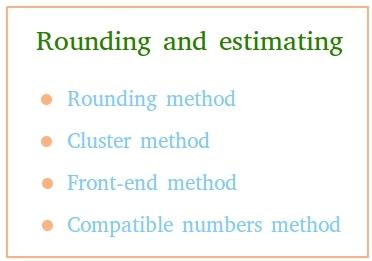Rounding And Estimation Basics

Rounding and estimation are very important skills not only in math but in your everyday life as well.

The truth is that rounding is a very handy tool in the business world when you are working with amounts. After all, using rounded numbers make a lot easier to work with numbers.

Discover the best round up and down calculators.

However, math also puts estimation at your disposal. Ultimately, an estimation gives you an idea about the possible answer without doing a lot of work. The reality is that when you need to know the right answer, you have to solve the problem. However, in daily life, estimation is a great way for giving intelligent guesses about possible amounts. You might see a pile of boxes and estimate that there are about 2,500 toys. It’s okay if the actual number is 2,479. You were close enough for a quick idea.

Rounding Basics

As you probably already know, in math, we can round numbers up and down.

Rounding up happens when you have a value that is half or greater of the amount you are rounding. Let’s say you want to round to the nearest ten. If you were given the value 26, you would round up because 6 is greater than half of ten (5). If you were given 25, you would also round up.

Round your numbers yo the nearest thousand.

Rounding down happens when the value is less than half. Using the same examples, if you were given the number 24 you would round down because the four is less than five. Even if you were given the wacky decimal 24.9999, you would still round down because 4.9999 is less than five. Here are some more examples:

Round 34 to the nearest 10: 30

Round 678 to the nearest 10: 680

Round 494 to the nearest 100: 500

Round 627 to the nearest 100: 600

Round 5,872 to the nearest 1,000: 6,000

Round 8,452 to the nearest 1,000: 8,000

Check out our nearest tens calculator.

Estimation Basics

Simply put, estimation is a process that is based on rounding numbers.

Estimation is commonly used when you are doing arithmetic with long numbers.

If you had the problem 567 + 248 and you only needed a close answer, you could estimate. That example might have you changing the values to 600 + 200 or 570 + 250.

Looking to round numbers to the nearest tenth?

Your first step was to round off both numbers to a specific place, and then you do the math. Your answer would be an estimation of the actual answer. You need to remember that estimation can give you answers that are very different from the actual answer. In our example, you might have estimated 800 or 820. With the actual answer of 815, you can see how the estimations were a bit off. Here are two more examples:

237 + 469 = ?

Rounding to 10: 240 + 470 = 710

Rounding to 100: 200 + 500 = 700